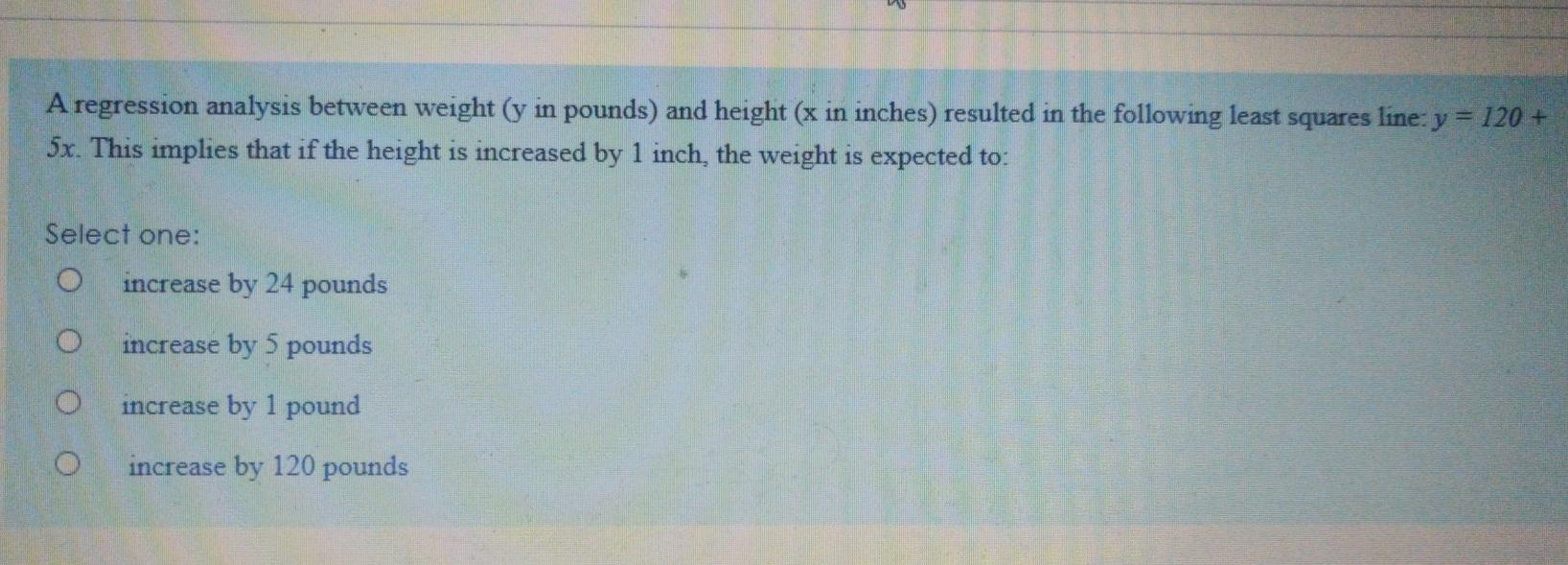# Question Solved1 Answerneed 100 percent perfect answer just answer please start solving quickly i will rate positive if answer is correct A regression analysis between weight (y in pounds) and height (x in inches) resulted in the following least squares line: y = 120 + 5x. This implies that if the height is increased by 1 inch, the weight is expected to: Select one: increase by 24 pounds o increase by 5 pounds increase by 1 pound O increase by 120 poundsMIEWCJ The Asker · Probability and Statisticsi will rate positive if answer is correct

Transcribed Image Text: A regression analysis between weight (y in pounds) and height (x in inches) resulted in the following least squares line: y = 120 + 5x. This implies that if the height is increased by 1 inch, the weight is expected to: Select one: increase by 24 pounds o increase by 5 pounds increase by 1 pound O increase by 120 pounds
More
Transcribed Image Text: A regression analysis between weight (y in pounds) and height (x in inches) resulted in the following least squares line: y = 120 + 5x. This implies that if the height is increased by 1 inch, the weight is expected to: Select one: increase by 24 pounds o increase by 5 pounds increase by 1 pound O increase by 120 pounds